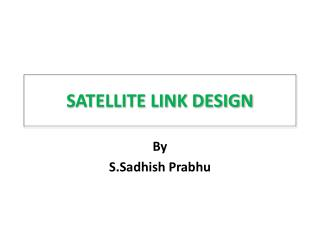Download Presentation# SATELLITE LINK DESIGN - PowerPoint PPT Presentation

SATELLITE LINK DESIGN. By S.Sadhish Prabhu. INTRODUCTION . Cost to build and launch a GEO satellite is about 25,000 dollars per kg Weight is the most critical factor in any design Dimension of the satellite : dia must be less than 3.5m Antennas are the limiting factor .I am the owner, or an agent authorized to act on behalf of the owner, of the copyrighted work described.
Download Presentation## SATELLITE LINK DESIGN

An Image/Link below is provided (as is) to download presentation

Download Policy: Content on the Website is provided to you AS IS for your information and personal use and may not be sold / licensed / shared on other websites without getting consent from its author.While downloading, if for some reason you are not able to download a presentation, the publisher may have deleted the file from their server.

- - - - - - - - - - - - - - - - - - - - - - - - - - E N D - - - - - - - - - - - - - - - - - - - - - - - - - -
Presentation Transcript

2. INTRODUCTION • Cost to build and launch a GEO satellite is about 25,000 dollars per kg • Weight is the most critical factor in any design • Dimension of the satellite : dia must be less than 3.5m • Antennas are the limiting factor

3. Factors influencing system design Weight of the satellite is driven by two factors • The number and the output power of he transponder on the satellite (requires large power from solar cells which in turn increases the weight ) • Weight of the station keeping fuel

4. Factors influencing system design • The choice of frequency band • Atmospheric propagation effects • Multiple access techniques

5. Performance objective • Bit error rate (BER) in a digital link • Signal-to-noise ratio (S/N) in an analog link • BER or S/N is determined by Carrier - to- noise ration (C/N) at the input of the demodulator in the receiver • C/N > 6 dB Measured in base band channel

6. Basic transmission theory Objective : Calculation of the power received by an earth station from satellite transmitter Two approaches for calculating : • Use of flux density • Link equation (Friis transmission equation )

7. Isotropic Radiator • Consider an Isotropic Source radiating Pt Watts uniformly into free space. • At distance R, the area of the spherical shell with center at the source is 4pR2 • Flux density at distance R is given by W/m2 Equ 4.1

8. Isotropic Radiator Isotropic Source Distance R Pt Watts Surface Area of sphere = 4pR2encloses Pt. W/m2 Power Flux Density:

9. Antenna Gain • We need directive antennas to get power to go in wanted direction. • Defined as the ratio of power per unit solid angle radiated in a direction to the average power radiated per unit solid angle (Eqn 4.2) • P() is variation of power with angle. • G() is gain at the direction . • P0 is total power transmitted. • sphere = 4p solid radians

10. Antenna Gain • Antenna has gain in every direction! • Usually “Gain” denotes the maximum gain of the antenna. • The direction of maximum gain is called “boresight”. • Gain is a ratio: • It is usually expressed in Decibels (dB) G [dB] = 10 log10 (G ratio)

11. Flux density The flux density in the direction of the antenna boresight at distance R meter is W/m2

12. EIRP (Pt*Gt) • An isotropic radiator is an antenna which radiates in all directions equally • Antenna gain is relative to this standard • Antennas are fundamentally passive • No additional power is generated • Gain is realized by focusing power • Similar to the difference between a lantern and a flashlight • Effective Isotropic Radiated Power (EIRP) is the amount of power the transmitter would have to produce if it was radiating to all directions equally • Note that EIRP may vary as a function of direction because of changes in the antenna gain vs. angle

13. EIRP Receiver Isotropic Source Incident flux disunity, F Received power Pt Pt Watts Receiving antenna area , A gain Gt R For an ideal receiving antenna with an aperture area of Am2, Pr= FA

14. EIRP • A antenna with physical aperture area of Arm2 will not deliver the power • Thus the efficiency is reduced • It is descried by using effective aperture Ae Ae = ηAr (4.5) Where η – aperture efficiency of the antenna Thus (4.6) Pr = 2

15. Fundamental of antenna theory (4.7) 2 Sub Ae in (4.6) 2 This expression is called as the Friis transmission equation

16. Contd.. (4.9) In decibel term (4.10) Where, EIRP = 10 log10 (PtGt)dBW Gr = 10 log10 dB Lp – path loss = 20 log10 dB 2

17. In general Pr = EIRP+Gr-Lp-La-Lta-LradBW(4.11) Where La = attenuation in atmosphere Lta= losses associated with transmitting antenna Lra = losses associated with receiving antenna

18. Reference of dB

19. Problem # 1 A satellite at a distance of 40,000km from a point on the earth’s surface radiates a power of 10W from an antenna with a gain of 17 dB in the direction of the observer, find the flux density at the receiving point, and the power received by an antenna at this point with an effective area 10m2

20. Problem # 2 • A satellite operates at a frequency of 11 GHz. The receiving antenna has a gain of 52.3 dB, Find the received power.

21. Answer -126dbW for both questio Note: The received power is commonly called as carrier power,C Because, Satellites use FM (Anlog transmission )or PM (digital transmission) In both modulation the carrier is not changed So, C=Pr